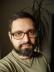Square Secret Code

The square secret code mechanism first removes any space from the original message. Then it lays down the message in a row of 8 columns. The coded message is then obtained by reading down the columns going left to right.

For example, the message is “The quick brown fox jumps over the lazy dog”. The code message would be as below:

tbjrd hruto eomhg qwpe unsl ifoa covz kxey

#!/usr/bin/perl
use warnings;
use strict;

use Test::More tests => 1;
is square_secret_code('The quick brown fox jumps over the lazy dog'),
'tbjrd hruto eomhg qwpe unsl ifoa covz kxey',
'encode';

Let’s use a regex to extract groups of 8 letters from the message. Then split each group into individual letters and append each of them to a string corresponding to an output word.

use Syntax::Construct qw{ /r // };

sub square_secret_code {
my (\$message) = @_;
my @code = ("") x 8;
for my \$group (\$message =~ s/\s//gr =~ m/(.{1,8})/g) {
\$code[\$_] .= (split //, \$group)[\$_] // "" for 0 .. 7;
}
return join ' ', @code
}

The test fails, though. Although no such transformation was mentioned, the code text is all lowercase. We can fix it easily by replacing \$message by lc(\$message) in the for loop line.

Note how the /r modifier makes it possible to remove whitespace and match the groups of eight characters tersely. The “defined or empty” part is there to prevent uninitialised warnings.

Source Dumper

Write a script that dumps its own source code. For example, say, the script name is ch-2.pl then the following command should return nothing.
\$ perl ch-2.pl | diff - ch-2.pl

Perl provides a special file handle assigned to the source of the running code. It’s called DATA and it’s created as soon as Perl compiles the special __DATA__ keyword. The filehandle points to the line after the keyword, but we can use seek to rewind it to the beginning of the file:

#!/usr/bin/perl
use warnings;
use strict;

seek *DATA, 0 , 0;
print <DATA>;
__DATA__

But the task can be solved in a completely different way. There’s a special category of programs called "quines" (named after Willard Van Orman Quine). Their sole purpose is to output their source without reading it. I was introduced to the idea several decades ago, the first quine I’ve ever seen was written in Pascal and I still keep a copy of it (tested in recent FreePascal; the trailing whitespace is part of the source!) I don’t know who the original author was, let me know if you do. Here it is in its miraculous beauty:

program t(output);
const d=40;
p=27;
z=8;
var a:array[11..10+p,1..d] of char;
r:11..10+p;
i:1..d;
begin
a:='program t(output);                      ';
a:='const d=40;                             ';
a:='      p=27;                             ';
a:='      z=8;                              ';
a:='var a:array[11..10+p,1..d] of char;     ';
a:='    r:11..10+p;                         ';
a:='    i:1..d;                             ';
a:='begin                                   ';
a:='for r:=11 to 10+z do                    ';
a:=' begin                                  ';
a:='  for i:=1 to d do write(a[r,i]);       ';
a:='  writeln                               ';
a:=' end;                                   ';
a:='for r:=11 to 10+p do                    ';
a:=' begin                                  ';
a:='  write(''a['',r,'']:='''''');                ';
a:='  for i:=1 to d do                      ';
a:='   if a[r,i]='''''''' then write('''''''''''')    ';
a:='                  else write(a[r,i]);   ';
a:='  writeln('''''';'')                        ';
a:=' end;                                   ';
a:='for r:=11+z to 10+p do                  ';
a:=' begin                                  ';
a:='  for i:=1 to d do write(a[r,i]);       ';
a:='  writeln                               ';
a:=' end                                    ';
a:='end.                                    ';
for r:=11 to 10+z do
begin
for i:=1 to d do write(a[r,i]);
writeln
end;
for r:=11 to 10+p do
begin
write('a[',r,']:=''');
for i:=1 to d do
if a[r,i]='''' then write('''''')
else write(a[r,i]);
writeln(''';')
end;
for r:=11+z to 10+p do
begin
for i:=1 to d do write(a[r,i]);
writeln
end
end.

When I started programming in Perl, I tried to write a similar program in it. It was much shorter, as Perl has more ways how to quote strings, and I shortened it even more over the years. You can find much shorter Perl quines on the Internet, but this one is precious to me as it’s my own invention:

\$_=q!print'\$_=q*'.\$_.'*;';s/\52/\41/g;print!;
print'\$_=q!'.\$_.'!;';s/\52/\41/g;print

The whole code should be placed on one line without a final newline or any other whitespace.

The idea is the same as in the Pascal example: prepare the code in a variable, then print it handling the quoting characters in a special way so they are escaped in the output in the same way as in the code.I blog about Perl.